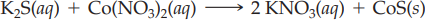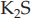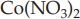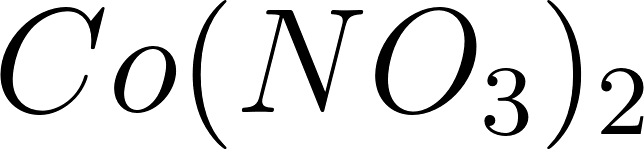×
Get Full Access to Introductory Chemistry - 5 Edition - Chapter 13 - Problem 92p
Get Full Access to Introductory Chemistry - 5 Edition - Chapter 13 - Problem 92p

×

# Consider the reaction:K2S(aq) + Co(NO3)2(aq) ? 2 KNO2(aq) + CoS(s)What volume of 0.225 MISBN: 9780321910295 34

## Solution for problem 92P Chapter 13

Introductory Chemistry | 5th Edition

• Textbook Solutions
• 2901 Step-by-step solutions solved by professors and subject experts
• Get 24/7 help from StudySoup virtual teaching assistantsIntroductory Chemistry | 5th Edition

4 5 1 384 Reviews
11
4
Problem 92P

Problem 92P

Consider the reaction:

K2S(aq) + Co(NO3)2(aq) → 2 KNO2(aq) + CoS(s)

What volume of 0.225 M K2S solution is required to completely react with 175 mL of 0.115 M Co(NO3)2?

Step-by-Step Solution:

Problem 92P

Consider the reaction:What volume of 0.225 Msolution is required to completely react with 175 mL of 0.115 M?

Step by Step Solution

Step 1 of 3

The given chemical reaction is as follows.From the above balanced chemical reaction one moles ofis treated with one moles ofto form two moles ofand.

Molarity can be calculated by the following formula...................(1)

Rearrange the formula (1)..........(2)

Step 2 of 3

Step 3 of 3

##### ISBN: 9780321910295

Unlock Textbook Solution